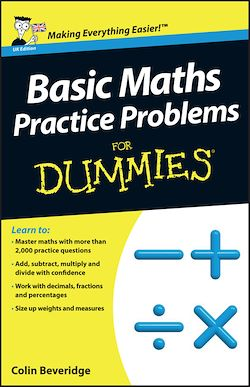384 Pages
English

# Basic Maths Practice Problems For Dummies

-Description

Learn to:

• Master maths with more than 2,000 practice questions
• Add, subtract, multiply and divide with confidence
• Work with decimals, fractions and percentages
• Size up weights and measures

Fun, friendly coaching and all the practice you need to tackle maths problems with confidence and ease

In his popular Basic Maths For Dummies, professional maths tutor Colin Beveridge proved that he could turn anyone – even the most maths-phobic person – into a natural-born number cruncher. In this book he supplies more of his unique brand of maths-made-easy coaching, plus 2,000 practice problems to help you master what you learn. Whether you're prepping for a numeracy test or an employability exam, thinking of returning to school, or you'd just like to be one of those know-it-alls who says, 'Oh, that's easy!' about any maths problem that comes your way, this book is for you.

• Master basic arithmetic, fast – in no time, solving addition, subtraction, multiplication and division problems will seem as easy as tying your shoes
• Face down fractions – you'll never again feel shy around fractions, decimals, percentages and ratios
• Juggle weights and measures like a pro – whether it's a question of how much it weighs, how long (or far) it is, or how much it costs, you'll never be at a loss for an answer
• Make shapes your playthings – circles, squares, triangles and rectangles – you'll measure them, draw them and manipulate them with ease

Open the book and find:

• 2,000 pencil-and-paper practice problems
• The keys to mastering addition, subtraction, multiplication and division
• The lowdown on fractions, decimals and percentages
• How to handle weights, measures and money problems
• How to read charts, tables and graphs at a glance

Subjects

##### Classwork

Informations